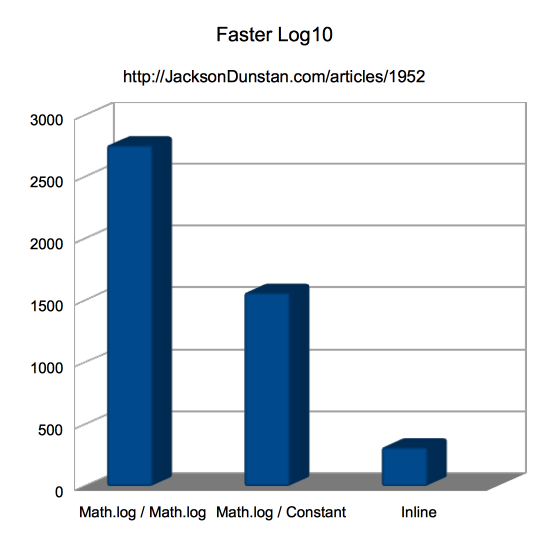Today’s article is quick and to the point: when you need to take the base 10 logarithm of an integer you can speed this up by about 8x. Read on for the technique and save some CPU cycles!

There is no function in the `Math` class to compute a base 10 logarithm. `Math.log` computes the base 2 logarithm, but you have to divide by `Math.log(base)` to convert this result to the base you want. Here’s an example for base 10:

```result = Math.log(input); // log base 2
result = Math.log(input) / Math.log(10); // log base 10```

But `Math.log(10)` is a constant, so you can cache this and only do one static function call on `Math`:

```LOG_10 = Math.log(10); // just one time
// ...
result = Math.log(input) / LOG_10; // log base 10```

Now for the new approach. This approach relies on the fact that, for 32-bit integers, there are only a few possible integer results: 0-9. So why not simply use a conditional statement like this one?

```result = (input >= 1000000000) ? 9 : (input >= 100000000) ? 8 : (input >= 10000000) ? 7 :
(input >= 1000000) ? 6 : (input >= 100000) ? 5 : (input >= 10000) ? 4 :
(input >= 1000) ? 3 : (input >= 100) ? 2 : (input >= 10) ? 1 : 0;```

Here’s a performance test app to compare how fast these three run:

```package
{
import flash.display.*;
import flash.utils.*;
import flash.text.*;

public class FasterLogarithms extends Sprite
{
private var __logger:TextField = new TextField();
private function row(...cols): void
{
__logger.appendText(cols.join(",")+"\n");
}

public function FasterLogarithms()
{
stage.align = StageAlign.TOP_LEFT;
stage.scaleMode = StageScaleMode.NO_SCALE;

__logger.autoSize = TextFieldAutoSize.LEFT;

init();
}

private function init(): void
{
var beforeTime:int;
var afterTime:int;
var i:int;
var REPS:int = 100000000;
var absInt:int;
const LOG_10:Number = Math.log(10);

row("Method", "Time");

beforeTime = getTimer();
for (i = 0; i < REPS; ++i)
{
absInt = Math.log(i) / Math.log(10);
}
afterTime = getTimer();
row("Math.log / Math.log", (afterTime-beforeTime), absInt);

beforeTime = getTimer();
for (i = 0; i < REPS; ++i)
{
absInt = Math.log(i) / LOG_10;
}
afterTime = getTimer();
row("Math.log / Constant", (afterTime-beforeTime), absInt);

beforeTime = getTimer();
for (i = 0; i < REPS; ++i)
{
absInt = (i >= 1000000000) ? 9 : (i >= 100000000) ? 8 : (i >= 10000000) ? 7 :
(i >= 1000000) ? 6 : (i >= 100000) ? 5 : (i >= 10000) ? 4 :
(i >= 1000) ? 3 : (i >= 100) ? 2 : (i >= 10) ? 1 : 0;
}
afterTime = getTimer();
row("Inline", (afterTime-beforeTime), absInt);
}
}
}```

I ran this test app in the following environment:

• Flex SDK (MXMLC) 4.6.0.23201, compiling in release mode (no debugging or verbose stack traces)
• Release version of Flash Player 11.4.402.265
• 2.3 Ghz Intel Core i7
• Mac OS X 10.8.1

And here are the results I got:

Method Time
Math.log / Math.log 2760
Math.log / Constant 1567
Inline 317As expected, the first version is about twice as slow as the second due to calling `Math.log` twice as many times. It’s an easy optimization to simply cache the call to `Math.log(10)`. That said, the real optimization is when we only need to operate on integers and choose to inline the log base 10 computation. Here we get an additional 5x performance boost! The combination of not having to call a static function of the `Math` class and the lack of any float-to-integer conversion really helps out. So if you’ve got any log base 10 operations in your performance critical code, consider a switchover if you can live with an integer-only restriction.